TUTORIALS
TUTORIALS HOME

GENERAL MATH
NOTATION & METHODS OF PROOF
INDUCTION
COMPLEX NUMBERS
POLYNOMIALS

LINEAR ALGEBRA
VECTORS
SYSTEM OF LINEAR EQUATIONS
MATRICES
EIGENVALUES & EIGENVECTORS
ORTHOGONALITY
VECTOR SPACE
DISTANCE & APPROXIMATION

HOME
TESTS
TUTORIALS
SAMPLE PROBLEMS
COMMON MISTAKES
STUDY TIPS
GLOSSARY
APPLICATIONS
MATH HUMOUR

# Orthogonal Sets

Vectors v, u are orthogonal or perpendicular to each other if v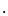u = 0 whenever v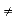u. We say a set of vectors {v1, v2, ... , vk} is an orthogonal set if for all vj and vi, vjvi = 0 where ij and i, j = 1, 2, ... , k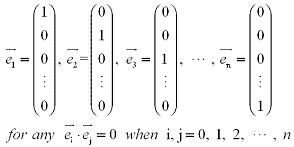We can show easily that the standard basis in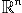is an orthogonal set

This is also true for any subset of the standard basis. Example one checks some other vectors for orthogonality.

Next we will look at some theorems that apply to orthogonal sets.

Theorem 1: If we have an orthogonal set {v1, v2, ... , vk} inthen vectors v1, v2, ... , vk are all linearly independent.
1 | Proof

Theorem 2: An orthogonal set of vectors v1, v2, ... , vn inform an orthogonal basis ofTheorem 3: Letting {v1, v2, ... , vk} be an orthogonal basis for a subspace ofand let w be any vector in the subspace. Then there exists scalars c1, c2, ... , ci, ... , ck such that:

w = c1v1+c2v2+ ... + civi+ ... +ckvk

# Examples:

1 | Check vectors for orthogonality
2 | Find coordinates with respect to a basis

# Orthonormal Sets

Next we will begin to explain the difference between an orthogonal and an orthonormal set.

The length or magnitude of v inis defined as ||v|| =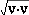A unit vector u inis a vector that has a length or magnitude of one. In other words: ||u|| = 1 or uu = 1

An orthonormal set is a set of vectors {v1, v2, ... , vk} which is orthogonal and every vector in the set is a unit vector. In other words for every vector vi in the set: ||vi|| = 1 and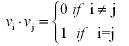where i = 1, 2, ... , k

It is quick and easy to obtain an orthonormal set for vectors from an orthogonal set of vectors, simply divide each vector by its length.

Theorem 4: If {v1, v2, ... , vk} is an orthonormal basis of a subspace ofthen for any vector w in the subspace, w can be expressed as:

w = (wv1)v1+ (wv2) v2+ ... + (wvk) vk

# Orthogonal Matrices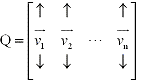An orthogonal matrix is simply a matrix whose columns are made up vectors form an orthogonal set. Letting Q be an orthogonal matrix we can then say that v1, v2, ... , vn (the columns of Q) form an orthongal set. Several theorems related to orthogonal matrices working with finding and maniputlating the transpose of the matrix. Therefore before getting into the theorems it would be benifical to first look at the properties of the transpose

Properties of the Transpose
1. If A is an n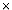m matrix and B is an mp matrix then (AB)T = BTAT
2. If an nn matrix A is invertible then so is AT and (AT)-1 = (A-1)T

Theorem 5: Let Q be and nn matrix. Then Q is orthogonal if and only if QTQ = In or equallently Q-1 = QT
5 | Proof

Theorem 6: Consider an nn matrix. Then Q then the following statements are equivallent:
1. Q is an orthogonal matrix
2. Q-1 = QT
3. QTQ = In
4. QxQy = xy for all x, y that exists in5. ||Qx|| = ||x|| which means, Q preserves length for every x in6 | Proof

Theorem 7: If Q is orthogonal then its rows form an orthonormal set of vectors and the columns of QT form an orthonormal set.
7 | Proof

# Examples:

3 | Check if a matrix is orthogonal

# Orthogonal Complements

The definition of orthogonal complement is similar to that of a normal vector. A vector n is said to be normal to a plane if it is orthogonal to every vector in that plane.

Letting W be a subspace ofand v be a vector inthen v is considered orthogonal to W if v is orthogonal to every vector that is contained in W. The orthogonal complement of W is the set of all vectors that are orthogonal to W. We will denote this set as Wpronounced the W perp.

W= {v in: vw = 0 for all w in W}

Theorem 8: Let W be a subspace of1. Wis a subspace of2. (W)= W
3. W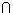W= {0}
8 | Proof

Theorem 9: Let A be an mn matrix. Then

(row(A))= null(A)     and     (col(A))= null(AT)

See the proof for a more detailed explaintion of this theorem.
9 | Proof

From this we see that a mn matrix A has four fundamental subspaces. The row(A), and the null(A) are the orthogonal complement inand the col(A), and the null(AT) are the orthogonal complement in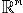.

# Examples

4 | Find the orthogonal complement of a subspace

# Orthogonal Projections

The definition of the projection of a vector v onto a onto a nonzero vector u was given in previous lectures by: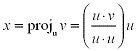Now consider a subspace W ofwith an orthogonal basis u1, u2 , ... , uk. Let v be any vector in, then the orthogonal projection of v onto W is defined as: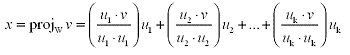If you have an orthonormal basis w1,w2, ... , wk of a subspace W and v is any vector inthen the orthonormal projection of v onto W is defined as: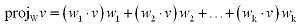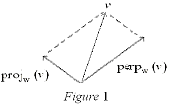Since the projw(v) is the projection of the vector v onto the subspace W we can also find a vector which is perpendicular or orthogonal to this projection. This vector is called perpw(v). We can decompose v as:

v = projw (v) + perpw(v)

The next theorem explains that you can always decompose a vector with respect to a subspace and its orthogonal complement.

Orthogonal Decomposition Theorem: Let a vector v and a subspace W exist in. Then there exists a unique vector w in W and win Wsuch that:

v = w + wTheorem 11: If W is a subspace of, then

dim W + dim W= n

11 | Proof

Rank Nullity Theorem: If A is an mn matrix, then

rank(A) + nullity(A)

12 | Proof

# Examples

5 | Find the orthogonal projection

# The Gram-Schmidt Process

As shown in the earlier examples, sometimes it is useful to have an orthogonal or orthonormal basis of a subspace of. There is a simple algorithm for constructing such a thing call the Gram-Schmidt Process.

If we had a subspace W ofthat has a two dimentional basis, x1 and x2 and we want to find an orthogonal basis w1 and w2 we use the Gram-Schmidt Process: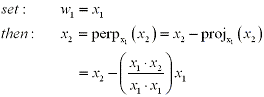If you have a subspace W inwhere W has a larger basis x1, x2, ... , xk follow the steps outlined in the algorithm in order to construct an orthogonal basis w1, w2, ... ,wk of W.
Algorithm: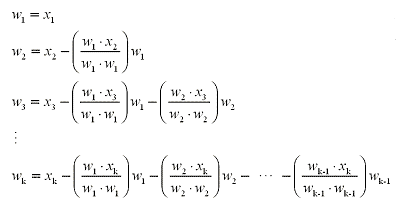# Examples

6 | Find an orthogonal basis for the subspace

# The QR Factorization

QR Factorization is a way of using the Gram-Schmidt Process to factor a mn matrix M with linearly independent columns. This factorization proves to be very useful to many other topics we will cover.

The QR Factorization Theorem: Consider an mn matrix M with linearly independent columns. Then M can be factored as M = QR, where Q is an mn matrix whose columns are orthonormal and R which is an invertible upper triangular matrix.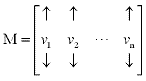Using QR Factorization is easy. Take an mn matrix M with linearly independent columns v1, v2, ... , vn. Then by using the Gram-Schmidt Process on the columns of M, find the orthonormal columns
w1, w2, ... , wn of Q.

Now each column vector v1, v2, ... , vn in M can be factored using the column vectors in Q.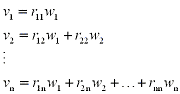The scalars r1i, r2i, ... ,rii (where i = 1, 2, ... , n) make up R. Then M can be broken down in the following QR Factorization.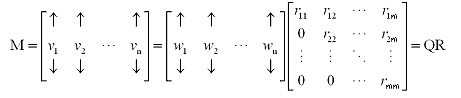And the following method can be used to find each scalar in R: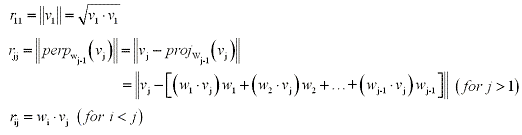Note: In the above method, wi where i = 1, 2, ... , n must be orthonormal vectors, not orthogonal.

# Examples

7 | Find the QR Factorization of the matrix

NOTE: This website has been designed for and tested for functionality on Internet Explorer 6.0, Netscape Navigator 7.2, Netscape Browser 8.0, and Mozilla Firefox 1.0. Other browsers may not display information correctly, although future versions of the abovementioned browsers should function properly.

COURSE HOMEPAGE
MATH 1046

FACULTY HOMEPAGES
Alex Karassev
Ted Chase